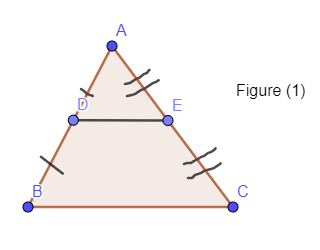Courses
Courses for Kids
Free study material
Free LIVE classes
More# In $\Delta ABC$, $D$ and $E$ are the mid-points of $AB$ and $AC$ respectively, then the area of $\Delta ADE$ is:A. $4\times$Area of $\Delta ABC$B. $\dfrac{1}{4}$Area of $\Delta ABC$C. $2\times$Area of $\Delta ABC$D. $\dfrac{1}{2}$Area of $\Delta ABC$

Last updated date: 25th Mar 2023
Total views: 306.9k
Views today: 8.83kVerified
306.9k+ views
Hint: Use the mid-point theorem to prove$\Delta ADE$and ABC as similar triangles. Now, use the property of similar triangles related to area and their sides. Now relate the area of $\Delta ADE$and$\Delta ABC$.

We can draw a diagram as per the given information asHere D is the midpoint of AB and E is the midpoint of AC and we need to determine the area of $\Delta ADE$in terms of area of $\Delta ABC$. As we already know the midpoint theorem which states that “Line joining midpoints of two sides of any triangle is parallel to the third side of the triangle and half of it.”

Hence, we can write equations from $\Delta ABC$as

$AD=\dfrac{1}{2}AB,AE=\dfrac{1}{2}AC,DE=\dfrac{1}{2}BC$

or$\dfrac{AD}{AB}=\dfrac{AE}{AC}=\dfrac{DE}{BC}=\dfrac{1}{2}\ldots \ldots (1)$
Now, in $\Delta ABC$and$\Delta ADE$, the ratio of sides of one triangle to another is equal to equation (1).

Hence $\Delta ABC$and $\Delta ADE$are similar to each other by SSS criteria. Now, property of similar triangles related with area and sides of triangles can be given as

“If two triangles are similar to each other, then the ratio of the area of the triangle is equal to the ratio of the corresponding square of their sides.”

Hence, if $\Delta ABC$and $\Delta ADE$are similar then, we have

$\dfrac{ar(ABC)}{ar(ADE)}=\dfrac{A{{B}^{2}}}{A{{D}^{2}}}=\dfrac{B{{C}^{2}}}{D{{E}^{2}}}=\dfrac{A{{C}^{2}}}{A{{E}^{2}}}\ldots \ldots (2)$

Now, from equation (1), we have

$\dfrac{AD}{AB}=\dfrac{AE}{AC}=\dfrac{DE}{BC}=\dfrac{1}{2}$

or$\dfrac{AB}{AD}=\dfrac{AC}{AE}=\dfrac{DE}{BC}=2$

Squaring of each term in above equation, we get

${{\left( \dfrac{AB}{AD} \right)}^{2}}={{\left( \dfrac{AC}{AE} \right)}^{2}}={{\left( \dfrac{DE}{BC} \right)}^{2}}=4\ldots \ldots (3)$

Now from equation (2) and (3), we get

$\dfrac{ar\left( \Delta ABC \right)}{ar\left( \Delta ADE \right)}=4$
On cross-multiplying, we get

$ar\left( \Delta ADE \right)=\dfrac{1}{4}ar\left( \Delta ABC \right)$
Hence, option B is the correct answer.

Note: Another approach for proving$\Delta ABC$and $\Delta ADE$similar is by mid-point theorem DE||BC.
Hence,
$\angle ADE=\angle ABC$ (Alternate angles)
$\angle AED=\angle ACB$ (Alternate angles)
$\angle A=\angle A$ (Common)
Hence, by AAA criteria $\Delta ABC$ is similar to $\Delta ADE$. Now apply the same approach for getting area
($\Delta ADE$).
One can go wrong while using the property of similar triangles related with area and sides. He/she may apply as the ratio of area of triangles should be equal to ratio of sides which is wrong. Ratio of sides is equal to ratio of squares of corresponding sides. Hence, be clear with this property and with midpoint property as well.
Using the mid-point theorem in solution is the key point of the question.